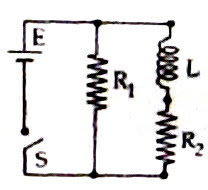## Friday, May 01, 2009

### All India Engineering/Architecture Entrance Examination (AIEEE) 2009 Question on Transients in LR Circuit

It is not by sitting still at a grand distance and calling the human race larvae that men are to be helped.

– Albert Einstein

The following question on transients in LR circuit was asked in the All India Engineering/Architecture Entrance Examination (AIEEE) 2009:An inductor of inductance L = 400 mH and resistors of resistances R1 = 2 Ω and R2 = 2 Ω are connected to a battery of emf 12 V as shown in the figure. The internal resistance of the battery is negligible. The switch S is closed at t = 0. The potential drop across L as a function of time is

(1) 12 e–5t V

(2) 6 e–5t V

(3) (12/t) e–3t

(4) 6(1e–t/0.2) V

The exponential growth of current I in an LR circuit is given by

I = I0 (1e–Rt/L) where I is the current at the instant t, I0 is the final maximum current equal to V/R where V is the voltage applied across the series LR combination and e is the base of natural logarithms. The potential drop across L at the instant t is L dI/dt

Therefore potential drop across L at the instant t = L (I0R/L) e–Rt/L = I0R e–Rt/L

In the present problem I0 = 6 A (since V = 12 volt and R = R2 = 2 Ω) and L = 400 mH = 0.4 H.

Therefore, the potential drop across L at the instant t = 6×2×e–2t/0.4 = 12 e–5t volt [Option (1)].

Let us try the following question involving transients in a CR circuits:

Suppose the inductor in the above question is replaced by a capacitor of capacitance 1000 μF. The switch S is closed at time t = 0. The charging current flowing through the capacitor as a function of time is

(1) 12 e–500t A

(2) 6 e–500t A

(3) 12e–t/1000 A

(4) 6(1e–t/500) A

The exponential growth of charge Q on a capacitor in a CR circuit is given by

Q = Q0 (1e–t/RC) where Q is the charge at the instant t, Q0 is the final maximum charge which is equal to CV. The charging current I flowing through the capacitor is given by

I = dQ/dt = (Q0/RC) e–t/RC = (CV/RC) e–t/RC = (V/R) e–t/RC

Here V = 12 volt, C = 1000 μF = 0.001 F and R = R2 = 2 Ω

Therefore, the charging current I flowing through the capacitor = 6× e–t/(2×0.001) = 6 e–500t A.

You will find a few useful multiple choice questions (with solution) on transients here at apphysicsresources. You can find more questions on transients in LR and CR circuits at other locations on the same site.